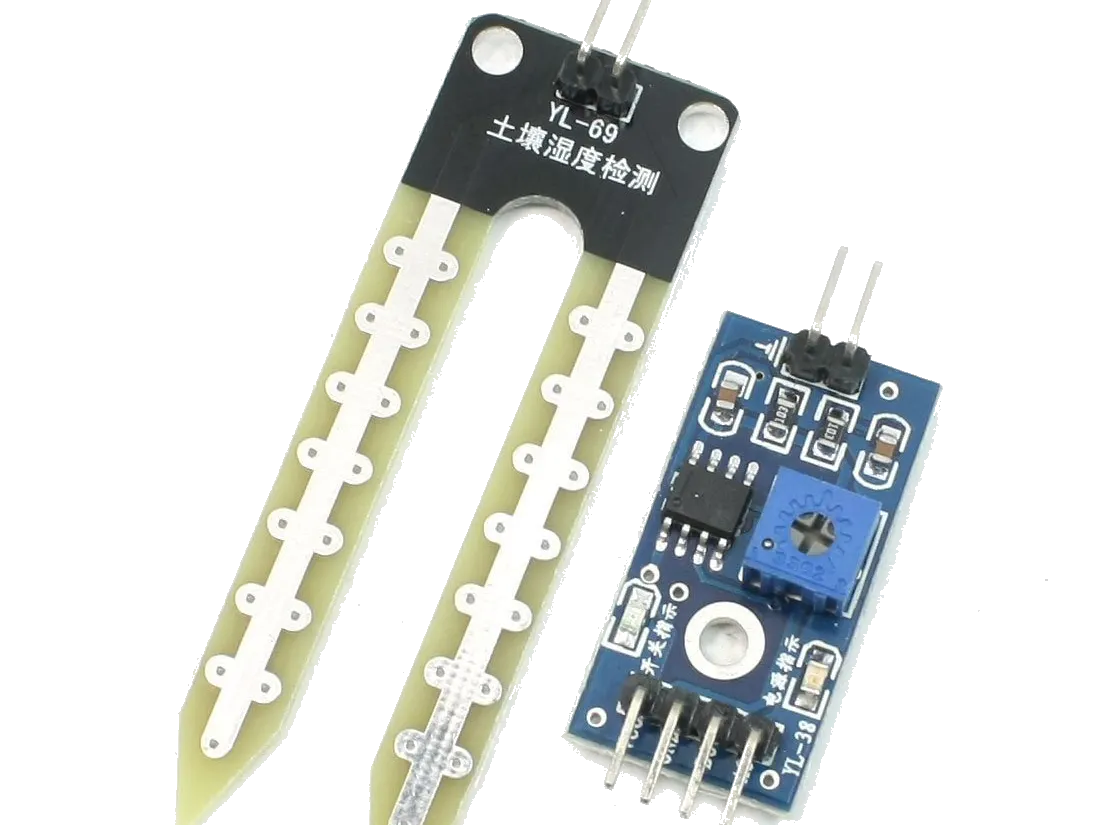# How to Use a Soil Moisture Sensor

This little project will show you how to use a soil moisture sensor.

• 56,606 views
• 51 respects

## Apps and online services

### Operation

The Soil Moisture Sensor measures soil moisture grace to the changes in electrical conductivity of the earth ( soil resistance increases with drought ).

The electrical resistance is measured between the two electrodes of the sensor.

A comparator activates a digital output when a adjutable threshold is exceeded.

### Code

At the time we start writing the code, we define 3 variables :

``````int sensorPin = A0;
``````

- The first one defines the analog pin of the Arduino

``````int sensorValue;
``````

- The second defines the analog value of the sensor read by the Arduino

``````int limit = 300;
``````
• The third defines a limit ( in this case if the sensorValue is larger than the limit, then a LED will light up )
• ----------------------------------------------------------------------------------------------------------------
``````void setup() {
Serial.begin(9600);
pinMode(13, OUTPUT);
}
``````

After, we initialize the serial monitor by indicating the number of baud ( here 9600 ) and also the thirteenth pin of the Arduino ( LED pin ) by indicating a current output.

-----------------------------------------------------------------------------------------------------------------

``````void loop() {
``````

We define the sensorValue as being the value read by the Arduino.

``````Serial.println("Analog Value : ");
Serial.println(sensorValue);
``````

We display the sensorValue on the serial monitor.

``````if (sensorValue<limit) {
digitalWrite(13, HIGH);
}
else {
digitalWrite(13, LOW);
}
delay(1000);
}
``````

This part of code allows to light up a LED if the sensorValue is smaller than the limit. Otherwise, the LED stays off.

### Connections

• Arduino --> Comparator

3V --> VCC

GND --> GND

A0 --> A0

• Comparator --> Sensor

+ --> +

- --> -

• Arduino --> LED

D13 --> +

GND --> -

Connect a resistance between + and - of the LED.

## Code

##### Soil Moisture Sensor Arduino
```int sensorPin = A0;
int sensorValue;
int limit = 300;

void setup() {
Serial.begin(9600);
pinMode(13, OUTPUT);
}

void loop() {

Serial.println("Analog Value : ");
Serial.println(sensorValue);

if (sensorValue<limit) {
digitalWrite(13, HIGH);
}
else {
digitalWrite(13, LOW);
}

delay(1000);
}
```

• 37,899 views
• 34 respects

#### Soil Moisture Sensor With LCD Display

Project tutorial by Patel Darshil

• 37,804 views
• 56 respects

#### The Quantified Cactus: An Easy Plant Soil Moisture Sensor

Project tutorial by Jen Looper

• 10,850 views
• 29 respects

#### Wireless Soil Moisture Probe with Helium and DFRobot

Project tutorial by Mark Phillips and Peter Main

• 15,165 views
• 33 respects

#### DIY Plant Moisture Sensor

Project tutorial by millerman4487

• 13,077 views